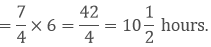# RD Sharma Class 7 ex 2.2 Solutions Chapter 2 Fractions

In this chapter, we provide RD Sharma Class 7 ex 2.2 Solutions Chapter 2 Fractions for English medium students, Which will very helpful for every student in their exams. Students can download the latest RD Sharma Class 7 ex 2.2 Solutions Chapter 2 Fractions Maths pdf, Now you will get step by step solution to each question.

## RD Sharma Solutions for Class 7 Chapter 2 FractionsEx 2.2Download PDF

Multiply

(i)  We have,

(ii)  We have,

(iii)  We have,

(iv) We have,

### Question: 2

Find the product:

(i)  We have,

(ii) We have,

(iv) We have,

Simplify:

(i)  We have,

(ii) We have,

(iii) We have,

Find:

(i) We have,

(ii) We have,

(iii) We have,

### Question: 5

Which is greater?

### Solution:

While comparing two fractions, when the numerators of both the fractions are same, then the denominator having higher value shows the fraction has lower value.

So, 6/14 is greater.

Therefore, 1/2 of 6/7 is greater.

### Question: 6

(i)  7/11 of 330

(ii) 5/9 of 108 meters

(iii) 3/7 of 42 litres

(iv) 1/12 of an hour

(v) 5/6 of an year

(vi) 3/20 of a Kg

(vii) 7/20 of a litres

(viii) 5/6 of a day

(ix)  2/7 of a week

### Solution:

(i)  We have,

(ii)  We have,

5/9 of 108 meters

= 5/9 × 108 meters

= 5 × 12 meters

60 meters

(iii) We have,

3/7 of 42 litres

= 3/7× 42 litres

= 3 × 6 litres

=18 litres

(iv) We have,

1/12 of an hour

An hour = 60 minutes

Therefore, 1/12 × 60 minutes

= 5 minutes

(v) We have,

5/6 of an year

I Year = 12 months

Therefore,

5/6 × 12 months

= 5 × 2 months

= 10 months

(vi) We have,

3/20 of a kg

1 Kg = 1000 gms

Therefore,

3/20 × 1000 gms

= 3 × 50 gms

= 150 gms

(vii) We have,

7/20 of a litre

1 litre = 1000 ml

Therefore,

7/20 × 1000 ml

= 7 × 50 ml

= 350 ml

(viii) We have,

5/6 of a day

I day = 24 hours

Therefore,

5/6 × 24 hours

= 5 × 4 hours

= 20 hours

(ix) We have,

2/7 of a week

I week = 7 days

Therefore,

2/7 × 7 days = 2 days

### Question: 7

Shikha plans 5 saplings in a row in her garden. The distance between two adjacent saplings is 3/4 m. Find the distance between first and last sapling.

### Solution:

There are 4 adjacent spacing for 5 saplings.

Given, the distance between two adjacent saplings is 3/4 m.

4 adjacent spacing for 5 saplings = 3/4 × 4 = 3 m

Therefore, the distance between first and last sapling is 3 m.

### Question: 8

Ravish reads 1/3 part of a book in one hour. How much part of the book will be read in 2(1/5) hours?

### Solution:

Let x be the full part of book.

Given, Ravish reads 1/3 part of a book in one hour

1 hour = (1/3) x

Part of the book will he read in 2(1/5) hours

### Question: 9

Lipika reads a book for 1(3/4) hours every day. She reads the entire book in 6 days. How many hours in all were required by her to read the book?

### Solution:

Given,

Time taken by Lipika to read a book per day = 1(3/4) = 7/4 hours

Time taken by Lipika to read a book for 6 days### Question: 10

Find the area of a rectangular park which is 41(2/3)m long and 18(3/5)m broad.

### Solution:

Area of a rectangular park = (length x breadth)

### Question: 11

If milk is available at Rs 17(3/4) per litre, find the cost of 7(2/5) litres of milk.

Given,

### Question: 12

Sharda can walk 8(1/3) km in one hour. How much distance will she cover in 2(2/5) hours.

### Solution:

Given,

Distance covered by Sharda in one hour = 25/3 km

### Question: 12

A sugar bag contains 30 kg of sugar. After consuming 2/3 of it, how much sugar is left in the bag

### Solution:

Given, A sugar bag contains 30 kg of sugar

After consuming 23 of it, the amount of sugar left in the bag

### Question: 14

Each side of a square is 6 (2/3)m long. Find its area.

Given,

### Question: 15

There are 45 students in a class and 3/5 of them are boys. How many girls are. there in the class?

### Solution:

Given,

There are 45 students in a class,

And 3/5 of them are boys.

Therefore, no of girls in the class = 45 –3/5 × 45

= 45 – 27

= 18

All Chapter RD Sharma Solutions For Class 7 Maths

I think you got complete solutions for this chapter. If You have any queries regarding this chapter, please comment on the below section our subject teacher will answer you. We tried our best to give complete solutions so you got good marks in your exam.